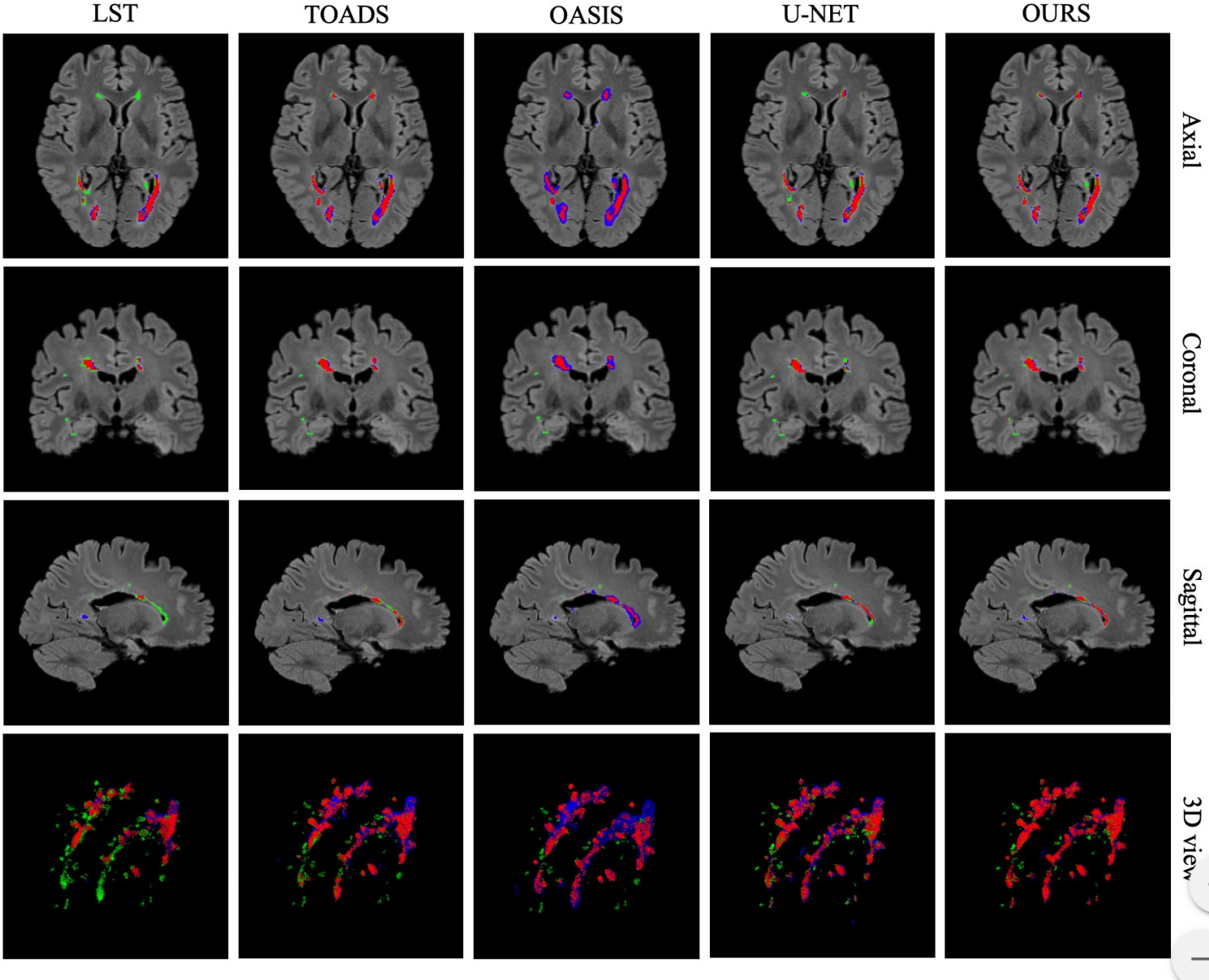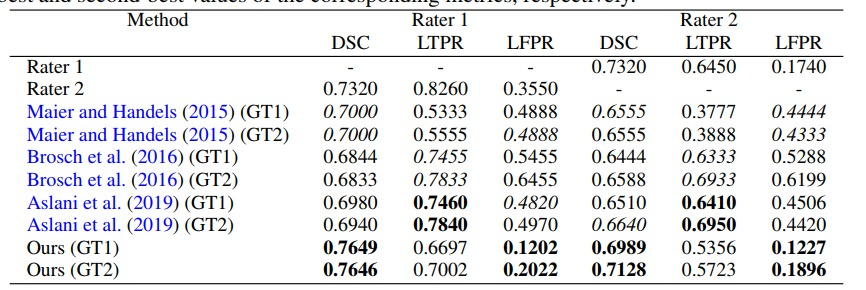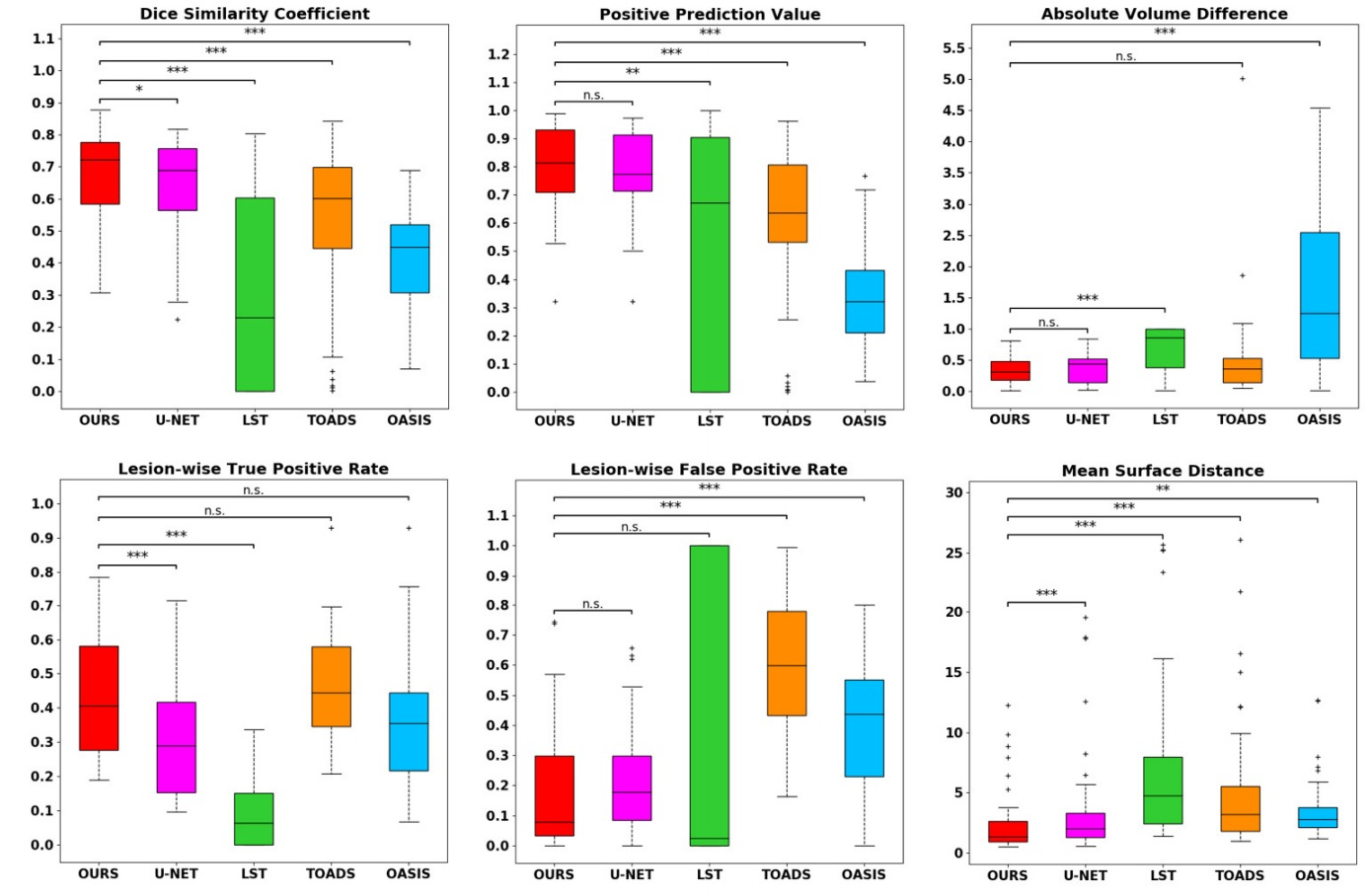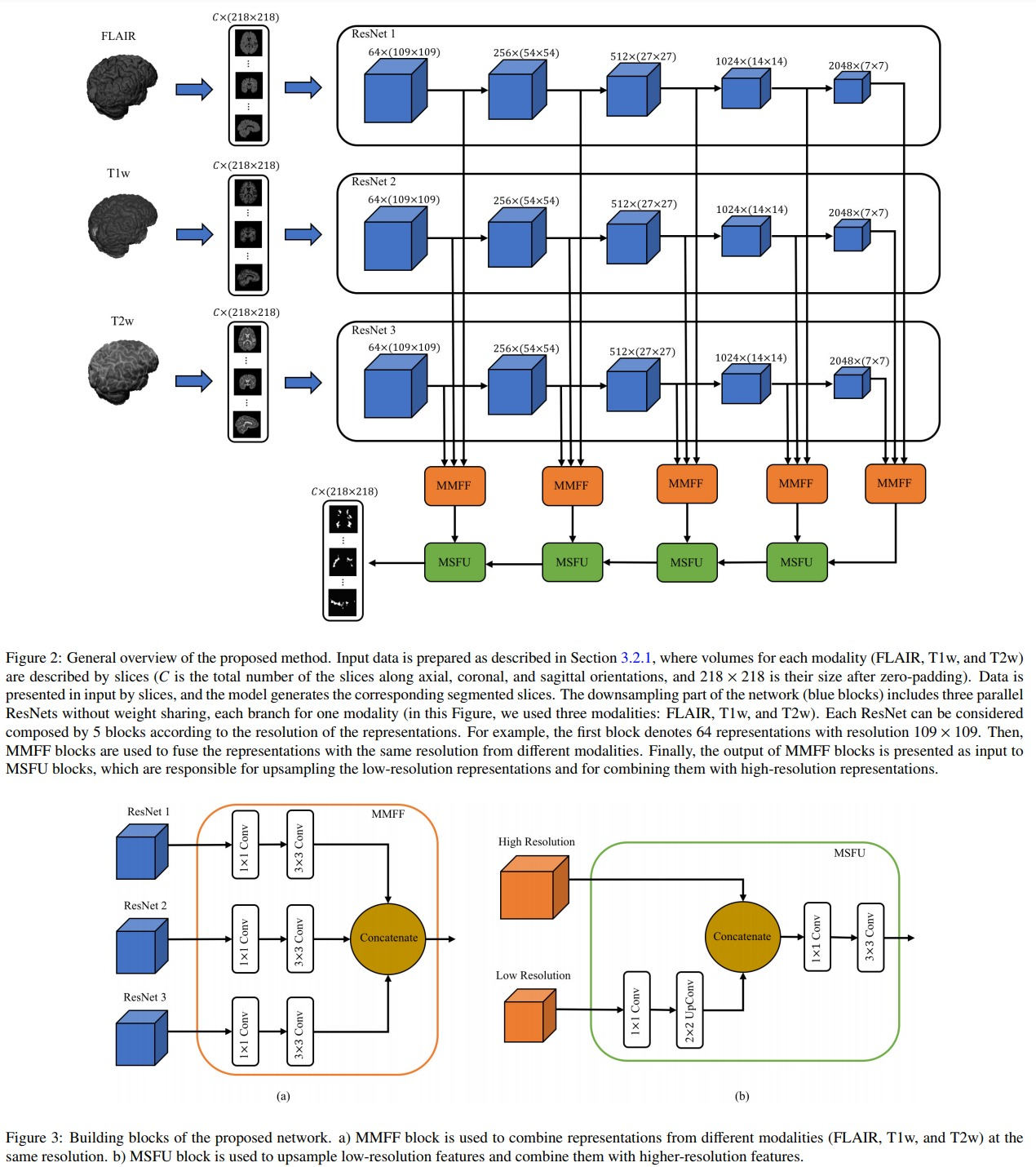## Introduction

This paper presents what seems to be the state-of-the-art multiple sclerosis segmentation method. This method has three main characteristics:

• A multi-modal ResNet encoder
• A multi-modal fusion at the skip connexion level
• A majority vote combination of sagittal, coronal and axial prediction.

## Proposed method

The proposed network is illustrated in Figure 2. The blue boxes are ResNet blocks, the orange boxes are Multi-Modality Feature Fusion (MMFF) blocks and the green boxes are Multi-Scale Feature Upsampling (MSFU) boxes. The loss implements a soft Dice function:

$DL = 1-\frac{2\sum_i g_ip_i}{\sum_i g_i^2 \sum_ip_i^2}$

where $$i$$ is a pixel index, $$p_i$$ is the predicted value for pixel $$i$$ (a probability value between 0 and 1) and $$g_i$$ is the groundtruth label for pixel $$i$$.

While the network processes 2D slices, it produces results for the axial, sagittal and coronal orientations. The three predicted values are then combined via a majority vote (c.f. the following figure )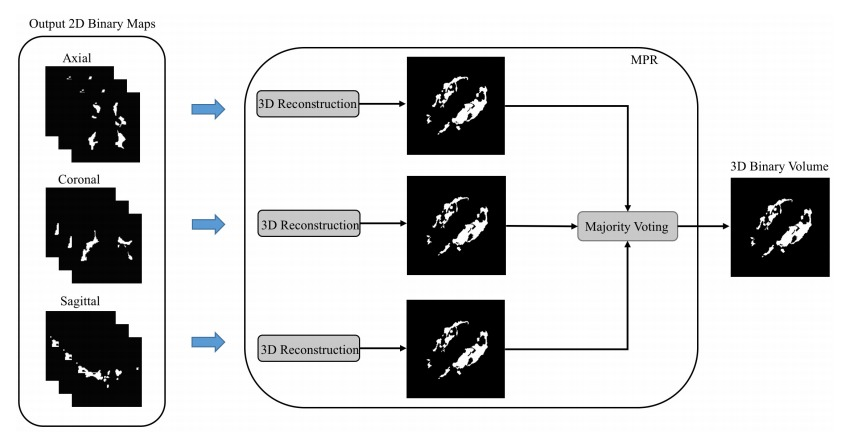## Results

They tested their method on the neuroimaging research unit (NRU) dataset which contains images of 37 patients. While their results are slightly better than those of a UNet, they are getting closer to the inter-observer variation. Unfortunately the paper has no ablation study so it is hard to quantify the benefit of every aspect of the method.